# A Comparison of maSAE to forestinventory

#### 2020-03-13, 13:16:56

In 2016, Andreas Hill published package forestinventory. We thought about merging the packages, but never actually got to it: maSAE is S4, forestinventory is S3, both of us are busy doing other stuff. So I am trying to at least compare functionality of both packages.

library("forestinventory")
library("maSAE")

# Setup

I need some helpers for checking and comparing results from maSAE and forestinventory:

clean <- function(x, which = NULL) {
if (identical(FALSE, which)) {
res <- as.matrix(unname(x[TRUE, TRUE]))
} else {
if (is.null(which)) {
if (all(c( "small_area", "prediction", "variance") %in% names(x))) {
res <- as.matrix(unname(x[TRUE, 1:3]))
} else {
res <- as.matrix(unname(x[["estimation"]][TRUE, c("area", "estimate", "g_variance")]))
}
} else {
res <- as.matrix(unname(x[TRUE, c(1, grep(which, names(x)))]))
}
}
return(res)
}

compare <- function(maSAE, forestinventory, message = NULL) {
if(! isTRUE(all.equal(clean(maSAE), clean(forestinventory),
check.attributes = FALSE))) {
message <- c("Differing results from maSAE and forestinventory: ",
message)
warning(message)
return(FALSE)
}
return(TRUE)
}

I use the grisons data set from forestinventory:

data(grisons, package = "forestinventory")

I define regression models for simple and cluster sampling:

formula.s0 <- tvol ~ mean # reduced model:
formula.s1 <- tvol ~ mean + stddev + max + q75 # full model
formula.clust.s1 <- basal ~ stade + couver + melange

# Two-Phase

Some data handling, true means are taken from forestinventorys vignette. :

truemeans.G <- data.frame(Intercept = rep(1, 4),
mean = c(12.85, 12.21, 9.33, 10.45),
stddev = c(9.31, 9.47, 7.90, 8.36),
max = c(34.92, 35.36, 28.81, 30.22),
q75 = c(19.77, 19.16, 15.40, 16.91))
rownames(truemeans.G) <- c("A", "B", "C", "D")

s1 <- grisons[grisons[["phase_id_2p"]] == 1, ]
s2 <- grisons[grisons[["phase_id_2p"]] == 2, ]
s12 <- rbind(s1, s2)
s12$s1 <- s12$phase_id_2p %in% c(1, 2)
s12$s2 <- s12$phase_id_2p == 2

tm <- truemeans.G
tm[["smallarea"]] <- row.names(tm)
tm[["Intercept"]] <- NULL

truemeans.G.partially <- truemeans.G[, -which(names(truemeans.G) %in% c("stddev", "mean"))]
tm.partially <- tm[, -which(names(tm) %in% c("stddev", "mean"))]

## No Exhaustive Auxiliary Information

This is the estimation given on bottom of page 15 of forestinventorys vignette:

summary(twophase(formula = formula.s1,
data = grisons,
phase_id = list(phase.col = "phase_id_2p", terrgrid.id = 2),
small_area = list(sa.col = "smallarea",
areas = c("A", "B","C", "D"),
unbiased = TRUE),
boundary_weights = "boundary_weights"
))
##
## Two-phase small area estimation
##
## Call:
## twophase(formula = formula.s1, data = grisons, phase_id = list(phase.col = "phase_id_2p",
##     terrgrid.id = 2), small_area = list(sa.col = "smallarea",
##     areas = c("A", "B", "C", "D"), unbiased = TRUE), boundary_weights = "boundary_weights")
##
## Method used:
## Extended pseudosynthetic small area estimator
##
## Regression Model:
## tvol ~ mean + stddev + max + q75 + smallarea
##
## Estimation results:
##  area estimate ext_variance g_variance  n1 n2 n1G n2G r.squared
##     A 391.9356     995.5602   1017.633 306 67  94  19 0.6526503
##     B 419.7231    1214.6053   1019.191 306 67  81  17 0.6428854
##     C 328.8600     916.2266   1036.791 306 67  66  15 0.6430018
##     D 373.9497    1272.7056   1110.245 306 67  65  16 0.6556178
##
## 'boundary_weight'- option was used to calculate weighted means of auxiliary variables

Now Im using both packages to make predictions:

maSAE <- predict(saObj(data = s12,
f = update(formula.s1, ~ . | smallarea),
auxiliaryWeights = "boundary_weights",
s2 = 's2')
)

forestinventory <- twophase(formula = formula.s1,
data = grisons,
phase_id = list(phase.col = "phase_id_2p", terrgrid.id = 2),
small_area = list(sa.col = "smallarea",
areas = c("A", "B","C", "D"),
unbiased = TRUE),
boundary_weights = "boundary_weights"
)

Both packages deliver the same results:

compare(maSAE, forestinventory, "two-phase, ext. pseudo sae")
##  TRUE

Now I benchmark them, calculating the small, synthetic and extended synthetic estimators:

wrap_two <- function(...) {
dots <- list(...)
dots$small_area$unbiased <- TRUE
ex <- do.call(twophase, dots)$estimation dots$psmall <- TRUE
small <- do.call(twophase, dots)$estimation dots$psmall <- FALSE
dots$small_area$unbiased <- FALSE
synth <- do.call(twophase, dots)$estimation cbind(ex[TRUE, c("estimate", "g_variance")], synth[TRUE, c("estimate", "g_variance")], small[TRUE, c("estimate", "g_variance")]) } mbmb <- microbenchmark::microbenchmark mb <- mbmb( forestinventory = wrap_two(formula = formula.s1, data = grisons, phase_id = list(phase.col = "phase_id_2p", terrgrid.id = 2), small_area = list(sa.col = "smallarea", areas = c("A", "B","C", "D"), unbiased = TRUE) ), maSAE = predict(saObj(data = s12, f = update(formula.s1, ~ . | smallarea), s2 = 's2' ))[, -1], check = "equivalent" ) maSAE seems a bit faster: print(mb) ## Unit: milliseconds ## expr min lq mean median uq max neval ## forestinventory 99.05001 102.38491 110.47926 107.19397 113.0910 203.1981 100 ## maSAE 46.88318 47.56144 53.10617 48.85753 54.1285 165.7101 100 microbenchmark:::autoplot.microbenchmark(mb) ## Coordinate system already present. Adding new coordinate system, which will replace the existing one.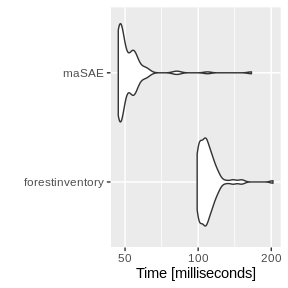## Full Exhaustive Auxiliary Information The estimation given on page 17 of forestinventorys vignette is (there are no boundary weights here): forestinventory <- twophase(formula = formula.s1, data = grisons, phase_id = list(phase.col = "phase_id_2p", terrgrid.id = 2), small_area = list(sa.col ="smallarea", areas = c("A", "B", "C", "D"), unbiased = TRUE), exhaustive = truemeans.G) summary(forestinventory) ## ## Two-phase small area estimation ## ## Call: ## twophase(formula = formula.s1, data = grisons, phase_id = list(phase.col = "phase_id_2p", ## terrgrid.id = 2), small_area = list(sa.col = "smallarea", ## areas = c("A", "B", "C", "D"), unbiased = TRUE), exhaustive = truemeans.G) ## ## Method used: ## Extended synthetic small area estimator ## ## Regression Model: ## tvol ~ mean + stddev + max + q75 + smallarea ## ## Estimation results: ## area estimate ext_variance g_variance n1 n2 n1G n2G r.squared ## A 372.6930 744.3658 696.5739 Inf 67 Inf 19 0.6526503 ## B 387.5116 693.8576 708.1105 Inf 67 Inf 17 0.6428854 ## C 334.8314 838.3953 801.4303 Inf 67 Inf 15 0.6430018 ## D 405.9667 940.3149 890.9536 Inf 67 Inf 16 0.6556178 Again, predictions from both packages are identical: maSAE <- predict(saObj(data = s12, f = update(formula.s1, ~ . | smallarea), s2 = 's2', smallAreaMeans = tm)) compare(maSAE, forestinventory, "two-phase, ext. sae") ##  TRUE The benchmarks again: mb <- mbmb( forestinventory = wrap_two(formula = formula.s1, data = grisons, phase_id = list(phase.col = "phase_id_2p", terrgrid.id = 2), small_area = list(sa.col ="smallarea", areas = c("A", "B", "C", "D"), unbiased = TRUE), exhaustive = truemeans.G), maSAE = predict(saObj(data = s12, f = update(formula.s1, ~ . | smallarea), s2 = 's2', smallAreaMeans = tm))[, -1], check = "equivalent") print(mb) ## Unit: milliseconds ## expr min lq mean median uq max neval ## forestinventory 76.81003 77.40702 81.11205 79.73291 84.09388 98.24379 100 ## maSAE 46.03427 46.36807 49.22237 46.82825 52.57498 72.98741 100 microbenchmark:::autoplot.microbenchmark(mb) ## Coordinate system already present. Adding new coordinate system, which will replace the existing one.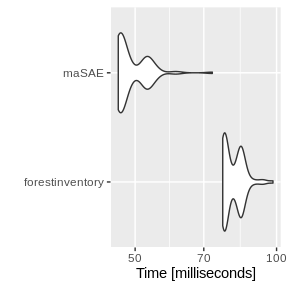# Three-Phase Some data handling, true means are taken from forestinventorys vignette. : truemeans.G <- data.frame(Intercept = rep(1, 4), mean = c(12.85, 12.21, 9.33, 10.45)) rownames(truemeans.G) <- c("A", "B", "C", "D") ## data adjustments s12_3p <- grisons[grisons[["phase_id_3p"]] %in% c(1,2), ] s0 <- grisons[grisons[["phase_id_3p"]] ==0 , ] s12_3p$s1 <- s12_3p$phase_id_3p %in% c(1, 2) s12_3p$s2 <- s12_3p$phase_id_3p == 2 s0$s1 <- s0$s2 <- FALSE predictors_s0 <- all.vars(formula.s0)[-1] predictors_s1 <- all.vars(formula.s1)[-1] eval(parse(text=(paste0("s0$",
setdiff(predictors_s1, predictors_s0),
" <- NA"))))
s012 <- rbind(s0, s12_3p)
tm <- truemeans.G
tm[["smallarea"]] <- row.names(tm)
tm[["Intercept"]] <- NULL

## No Exhaustive Auxiliary Information

The estimation given on page 23 of forestinventorys vignette is:

summary(threephase(formula.s0,
formula.s1,
data = grisons,
phase_id = list(phase.col = "phase_id_3p", s1.id = 1, terrgrid.id = 2),
small_area=list(sa.col = "smallarea", areas = c("A", "B", "C", "D"),
unbiased = TRUE),
boundary_weights = "boundary_weights"
))
##
## Three-phase small area estimation
##
## Call:
## threephase(formula.s0 = formula.s0, formula.s1 = formula.s1,
##     data = grisons, phase_id = list(phase.col = "phase_id_3p",
##         s1.id = 1, terrgrid.id = 2), small_area = list(sa.col = "smallarea",
##         areas = c("A", "B", "C", "D"), unbiased = TRUE), boundary_weights = "boundary_weights")
##
## Method used:
## Extended pseudosynthetic small area estimator
##
## Full Regression Model:
## tvol ~ mean + stddev + max + q75 + smallarea
##
## Reduced Regression Model:
## tvol ~ mean + smallarea
##
## Estimation results:
##  area estimate ext_variance g_variance  n0  n1 n2 n0G n1G n2G r.squared_reduced
##     A 395.1882    1901.2107  1858.2042 306 128 40  94  38  12         0.5454824
##     B 389.8329    1846.9952  1816.6552 306 128 40  81  34  11         0.5354637
##     C 321.9967     722.7413   763.0731 306 128 40  66  28   8         0.5282291
##     D 365.4938    2248.9395  1930.6881 306 128 40  65  28   9         0.5339996
##  r.squared_full
##       0.7242913
##       0.7171512
##       0.7172375
##       0.7268820
##
## 'boundary_weight'- option was used to calculate weighted means of auxiliary variables

Wait, the ext_variance differs, but thats a problem withforestinventory…

I make predictions omitting the boundary weights:

forestinventory <- threephase(formula.s0,
formula.s1,
data = grisons,
phase_id = list(phase.col = "phase_id_3p", s1.id = 1, terrgrid.id = 2),
small_area=list(sa.col = "smallarea", areas = c("A", "B", "C", "D"),
unbiased = TRUE),
boundary_weights = "boundary_weights"
)

maSAE <- predict(saObj(data = s012,
f = update(formula.s1, ~ . | smallarea),
s1 = 's1',
auxiliaryWeights = "boundary_weights",
s2 = 's2'))
## n(s0) >> n(s1) should hold, but you've given s1 resulting in n(s1)/n(s0) =  0.418300653594771
compare(maSAE, forestinventory, "three-phase, ext. pseudo sae")
##  TRUE

The benchmarks again:

wrap_three <- function(...) {
dots <- list(...)
dots$small_area$unbiased <- TRUE
ex <- do.call(threephase, dots)$estimation dots$psmall <- TRUE
small <- do.call(threephase, dots)$estimation dots$psmall <- FALSE
dots$small_area$unbiased <- FALSE
synth <- do.call(threephase, dots)$estimation cbind(ex[TRUE, c("estimate", "g_variance")], synth[TRUE, c("estimate", "g_variance")], small[TRUE, c("estimate", "g_variance")]) } mb <- mbmb( forestinventory = wrap_three(formula.s0, formula.s1, data = grisons, phase_id = list(phase.col = "phase_id_3p", s1.id = 1, terrgrid.id = 2), small_area=list(sa.col = "smallarea", areas = c("A", "B", "C", "D"), unbiased = TRUE) ), maSAE = predict(suppressMessages(saObj(data = s012, f = update(formula.s1, ~ . | smallarea), s1 = 's1', s2 = 's2')))[, -1], check = "equivalent") print(mb) ## Unit: milliseconds ## expr min lq mean median uq max ## forestinventory 157.81069 165.83821 174.25472 167.94399 174.37054 298.1265 ## maSAE 77.31124 78.72537 83.52233 83.15628 86.25088 116.0863 ## neval ## 100 ## 100 microbenchmark:::autoplot.microbenchmark(mb) ## Coordinate system already present. Adding new coordinate system, which will replace the existing one.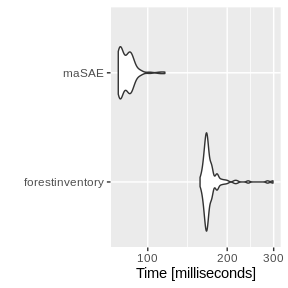# Partially Exhaustive Auxiliary Information Funny: forestinventory cant deal with partially exhaustive auxiliary information for two-phase sampling: try(twophase(formula = formula.s1, data = grisons, phase_id = list(phase.col = "phase_id_2p", terrgrid.id = 2), small_area = list(sa.col ="smallarea", areas = c("A", "B", "C", "D"), unbiased = TRUE), exhaustive = truemeans.G.partially)) ## Error in names(Z_bar_1G) <- colnames(design_matrix.s2) : ## 'names' attribute  must be the same length as the vector  predict(saObj(data = s12, f = update(formula.s1, ~ . | smallarea), s2 = 's2', smallAreaMeans = tm.partially)) ## small_area prediction variance psynth var_psynth psmall var_psmall ## 1 A 371.0402 712.3895 401.8953 287.3151 373.9803 1048.5674 ## 2 B 398.9159 944.4190 398.5562 303.4478 399.4579 996.5841 ## 3 C 330.4869 863.1605 334.5188 261.8389 330.6825 1104.9620 ## 4 D 384.7398 999.1490 345.1298 227.8379 380.9174 1203.8859 Whereas maSAE cant deal with partially exhaustive auxiliary information for three-phase sampling: summary(forestinventory <- threephase(formula.s0, formula.s1, data = grisons, phase_id = list(phase.col = "phase_id_3p", s1.id = 1, terrgrid.id = 2), small_area = list(sa.col = "smallarea", areas = c("A", "B", "C", "D"), unbiased = TRUE), exhaustive = truemeans.G)) ## ## Three-phase small area estimation ## ## Call: ## threephase(formula.s0 = formula.s0, formula.s1 = formula.s1, ## data = grisons, phase_id = list(phase.col = "phase_id_3p", ## s1.id = 1, terrgrid.id = 2), small_area = list(sa.col = "smallarea", ## areas = c("A", "B", "C", "D"), unbiased = TRUE), exhaustive = truemeans.G) ## ## Method used: ## Extended synthetic small area estimator ## ## Full Regression Model: ## tvol ~ mean + stddev + max + q75 + smallarea ## ## Reduced Regression Model: ## tvol ~ mean + smallarea ## ## Estimation results: ## area estimate ext_variance g_variance n0 n1 n2 n0G n1G n2G r.squared_reduced ## A 380.7982 1642.0551 1524.8061 Inf 128 40 Inf 38 12 0.5454824 ## B 368.8658 1501.2108 1530.6216 Inf 128 40 Inf 34 11 0.5354637 ## C 325.7081 640.2232 541.0235 Inf 128 40 Inf 28 8 0.5282291 ## D 389.3585 1961.1322 1753.9986 Inf 128 40 Inf 28 9 0.5339996 ## r.squared_full ## 0.7242913 ## 0.7171512 ## 0.7172375 ## 0.7268820 try(predict(saObj(data = s012, f = update(formula.s1, ~ . | smallarea), s2 = 's2', s1 = 's1', smallAreaMeans = tm))) ## n(s0) >> n(s1) should hold, but you've given s1 resulting in n(s1)/n(s0) = 0.418300653594771 ## Error in h(simpleError(msg, call)) : ## error in evaluating the argument 'object' in selecting a method for function 'predict': invalid class "saeObj" object: Got both partial true means and s1. There is no theoretical description for this so far! I do not see what three-phase partially exhaustive information would be. So: is partially exhaustive auxiliary information two- or three-phase? ## Is Partially Exhaustive Auxiliary Information Two- or Three-Phase? The (first) estimation given on page 22 of forestinventorys vignette is: extsynth_3p <- threephase(formula.s0, formula.s1, data = grisons, phase_id = list(phase.col = "phase_id_3p", s1.id = 1, terrgrid.id = 2), small_area = list(sa.col = "smallarea", areas = c("A", "B", "C", "D"), unbiased = TRUE), exhaustive = truemeans.G, boundary_weights = "boundary_weights" ) extsynth_3p$estimation
##   area estimate ext_variance g_variance  n0  n1 n2 n0G n1G n2G
## 1    A 382.6405    1642.0551  1518.7407 Inf 128 40 Inf  38  12
## 2    B 368.9013    1501.2108  1530.5759 Inf 128 40 Inf  34  11
## 3    C 325.3720     640.2232   543.2681 Inf 128 40 Inf  28   8
## 4    D 388.0325    1961.1322  1756.0906 Inf 128 40 Inf  28   9
##   r.squared_reduced r.squared_full
## 1         0.5454824      0.7242913
## 2         0.5354637      0.7171512
## 3         0.5282291      0.7172375
## 4         0.5339996      0.7268820
s12_3p$s1 <- NULL s12_3p$phase_id_2p <- NULL
s12_3p\$phase_id_3p <- NULL
maSAE <- predict(saObj(data = s12_3p,
f = update(formula.s1, ~ . | smallarea),
auxiliaryWeights = "boundary_weights",
s2 = 's2', smallAreaMeans = tm)
)
compare(maSAE, extsynth_3p, "three-phase, ext. sae")
##  TRUE

So this is a two-phase setup, forestinventory seems to need the three-phase setup (the reduced model) to identify the partially exhaustive part of the auxiliary information. The corresponding publication is

Daniel Mandallaz, Jochen Breschan, and Andreas Hill. New regression estimators in forest inventories with two-phase sampling and partially exhaustive information: a design-based Monte Carlo approach with applications to small-area estimation. In: Canadian Journal of Forest Research 43.11 (2013), pp. 1023-1031. doi: 10.1139/cjfr-2013-0181.

The benchmarks again:

mb <- mbmb(
forestinventory = wrap_three(formula.s0,
formula.s1,
data = grisons,
phase_id = list(phase.col = "phase_id_3p", s1.id = 1, terrgrid.id = 2),
small_area = list(sa.col = "smallarea", areas = c("A", "B", "C", "D"),
unbiased = TRUE),
exhaustive = truemeans.G),
maSAE = predict(suppressMessages(saObj(data = s12_3p,
f = update(formula.s1, ~ . | smallarea),
s2 = 's2', smallAreaMeans = tm)))[, -1],
check = "equivalent")
print(mb)
## Unit: milliseconds
##             expr       min        lq      mean    median        uq      max
##  forestinventory 147.60877 155.38166 170.62054 158.49756 178.25596 276.3504
##            maSAE  74.74929  76.93124  87.06018  83.05022  90.18082 139.8529
##  neval
##    100
##    100
microbenchmark:::autoplot.microbenchmark(mb)
## Coordinate system already present. Adding new coordinate system, which will replace the existing one.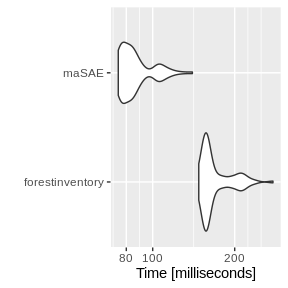# Cluster Sampling

I adapt data from maSAE to section 6.2 of forestinventorys vignette:

suppressWarnings(rm("s1" ,"s2", "s12"))
data("s1", "s2", package = "maSAE")
s12 <- bind_data(s1, s2)
s12[["g"]][is.na(s12[["g"]])] <- "a"
s12[["phase"]]  <-  s12[["phase1"]] + s12[["phase2"]]
maSAE <- predict(suppressMessages(saObj(data = s12, f = y ~x1 + x2 + x3 | g,
s2 = "phase2", cluster = "clustid")))
extpsynth.clust <- twophase(y ~x1 + x2 + x3,
data = s12,
cluster = "clustid",
phase_id = list(phase.col = "phase", s1.id = 1, terrgrid.id = 2),
small_area = list(sa.col = "g", areas = c("a", "b"),
unbiased = TRUE))
compare(maSAE, extpsynth.clust, "three-phase, ext. sae")
##  TRUE
mb <- mbmb(
forestinventory = clean(twophase(y ~x1 + x2 + x3,
data = s12,
cluster = "clustid",
phase_id = list(phase.col = "phase", s1.id = 1, terrgrid.id = 2),
small_area = list(sa.col = "g", areas = c("a", "b"),
unbiased = TRUE))),
maSAE = clean(predict(suppressMessages(saObj(data = s12, f = y ~x1 + x2 + x3 | g,
s2 = "phase2", cluster = "clustid")))),

check = "equivalent")
print(mb)
## Unit: milliseconds
##             expr       min        lq      mean    median        uq       max
##  forestinventory 100.31116 103.29630 111.21847 107.85945 113.14509 228.92764
##            maSAE  41.25872  42.04113  45.94833  44.13972  48.30483  72.41759
##  neval
##    100
##    100
microbenchmark:::autoplot.microbenchmark(mb)
## Coordinate system already present. Adding new coordinate system, which will replace the existing one.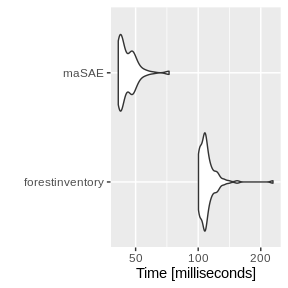Now I use the example given in section 6.2 of forestinventorys vignette:

  data("zberg", package = "forestinventory")
forestinventory <- forestinventory::twophase(
formula = basal ~ stade + couver + melange, data = zberg,
phase_id = list(phase.col = "phase_id_2p", terrgrid.id = 2),
cluster = "cluster",
small_area = list(
sa.col = "ismallold", areas = c("1"),
unbiased = TRUE
)
)
## Warning: At least one terrestrial cluster not entirely included within the small area 1.
## Zero mean residual assumption for small area maybe violated.
## Check mean_Rc_x_hat_G and consider alternative estimator 'psmall'
  s1 <- zberg[zberg[["phase_id_2p"]] == 1, ]
s2 <- zberg[zberg[["phase_id_2p"]] == 2, ]
s12 <- rbind(s1, s2)
s12[["s1"]] <- s12[["phase_id_2p"]] %in% c(1, 2)
s12[["s2"]] <- s12[["phase_id_2p"]] == 2
object <- maSAE::saObj(
data = s12,
f = basal ~ stade + couver + melange | ismallold,
s2 = "s2",
cluster = "cluster"
)
## include is NULL, automatically adding it as TRUE to data.
  maSAE <- maSAE::predict(object)
compare(maSAE[2,], forestinventory, "clustered, ext. sae")
## Warning in compare(maSAE[2, ], forestinventory, "clustered, ext. sae"):
## Differing results from maSAE and forestinventory: clustered, ext. sae
##  FALSE

Obviously, something went wrong. The difference to the previous example? zberg contains nominally scaled predictors. If I ignore the cluster design, both packages give the same result:

  forestinventory <- forestinventory::twophase(
formula = basal ~ stade + couver + melange, data = zberg,
phase_id = list(phase.col = "phase_id_2p", terrgrid.id = 2),
small_area = list(
sa.col = "ismallold", areas = c("1"),
unbiased = TRUE
)
)
object <- maSAE::saObj(
data = s12,
f = basal ~ stade + couver + melange | ismallold,
s2 = "s2",
)
maSAE <- maSAE::predict(object)
compare(maSAE[2,], forestinventory, "clustered, ext. sae")
##  TRUE

I do not know where that comes from. But since maSAE has very structured code compared to forestinventory (maSAE needs about 500 lines of code for its prediction functions, forestinventory more than 2200), I am biased to believe maSAE.

# Conclusion

• Both packages give mostly identical results, but different ones for clustered sampling designs with nominally scaled predictors.
• forestinventory views partially exhaustive auxiliary information as a three-phase setup, maSAE (following Mandallaz’ publications) uses a two-phase setup, but both packages give identical results after some data tweaking.
• maSAE seems to be a bit faster.
• forestinventory has global estimators implemented.